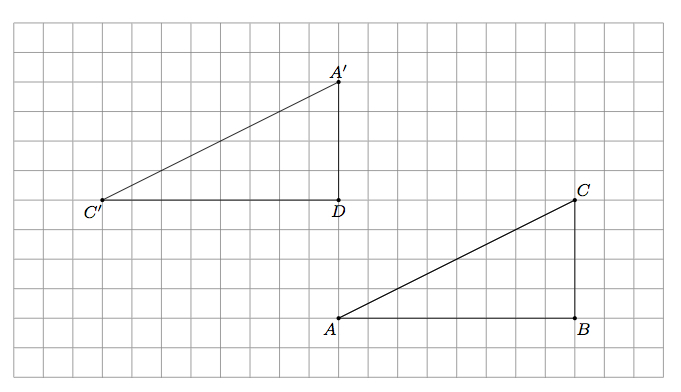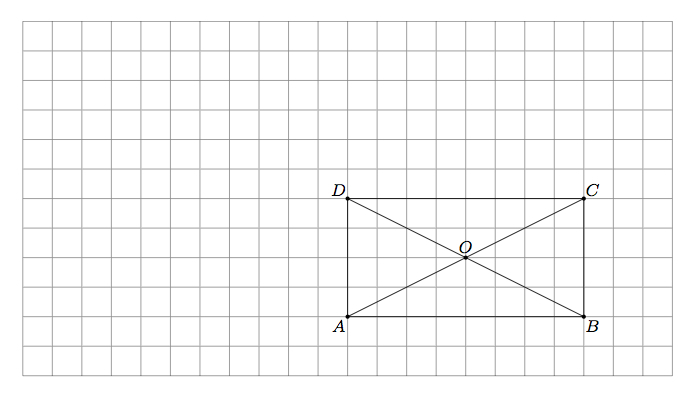# Cutting a rectangle into two congruent triangles

Alignments to Content Standards: 8.G.A.2

Below is a picture of rectangle $ABCD$ with diagonal $AC$.1. Draw the image of triangle $ACD$ when it is rotated $180^\circ$ about vertex $D$. Call $A'$ the image of point $A$ under the rotation and $C'$ the image of point $C$.
2. Explain why $\overleftrightarrow{DA'} \cong \overleftrightarrow{DA}$ and why $\overleftrightarrow{DC'}$ is parallel to $\overleftrightarrow{AB}$.
3. Show that $\triangle A'C'D$ can be translated to $\triangle CAB$. Conclude that $\triangle ACD$ is congruent to $\triangle CAB$.
4. Show that $\triangle ACD$ is congruent to $\triangle CAB$ with a sequence of translations, rotations, and/or reflections different from those chosen in parts (a) and (c).

## IM Commentary

This task shows the congruence of two triangles in a particular geometric context arising by cutting a rectangle in half along the diagonal. The teacher may wish to have students think about part (d) of the problem first before going through parts (a) through (c). The grid has been added to the background as a geometric aid and as a means of determining lengths of sides. For part (d), the grid may need to be extended depending on which translations, rotations, and reflections the students choose to use.

A good way of reasoning about this problem abstractly would be to observe that the triangles are both right triangles and the lengths of the sides that come together to form the right angles are $4$ and $8$ units respectively. So to show that they are congruent we just need to "align" the right angles and the sides with corresponding lengths. Some questions which teachers may wish to have students explore further are:

• Is it possible to show the congruence using only translations?
• Is it possible to show the congruence with a reflection and then a translation?
• Is it possible to show the congruence using only reflections?

The task is primarily intended for instructional purposes. Part (b) has been added explicitly to help students visualize how certain $180^\circ$ rotations influence a coordinate grid. Also part (d) has many possible answers, providing an ideal opportunity to have students share their (different) constructions with one another. If the teacher wishes to give students a chance to be creative and explore many different ways to transform $\triangle ACD$ to $\triangle CAB$ then part (d) alone can be used.

## Solution

1. Rotation by $180^\circ$ about $D$ takes any line through $D$ to itself but switches the orientation of the line. So, for example, line $\overleftrightarrow{DA}$ is taken to $\overleftrightarrow{DA}$ but the notions of up and down on this vertical line are reversed. So point $A$, which is four units below $D$ will be mapped to the point $A'$ which is four units above $D$. The points $D$ and $C$, on the other hand, lie on a horizontal line through $D$. So rotation by $180^\circ$ about $D$ has the effect of switching left and right on this line. Since $C$ lies $8$ units to the right of $D$, its image, $C'$, after rotating $180^\circ$ about $D$ will be the $8$ units to the left of $D$. This is pictured below:2. As explained in part (a), rotation by $180^\circ$ about $D$ maps any line through $D$ to itself, exchanging the two rays through $D$ which make up the line. This means that $\overleftrightarrow{DA'} = \overleftrightarrow{DA}$ since $\overleftrightarrow{DA'}$ is the rotation of line $\overleftrightarrow{DA}$ by $180^\circ$ about $D$. Similarly, $\overleftrightarrow{DC'} = \overleftrightarrow{DC}$. So $\overleftrightarrow{DC'}$ is a horizontal line of the grid, as is $\overleftrightarrow{AB}$, and so these two lines are parallel, remaining four units apart from one another.

3. To send $D$ to $B$ we need to translate by $8$ units to the right and $4$ units down. Translating by $8$ units to the right and $4$ units down also takes $C'$ to $A$ and $A'$ to $C$ as is seen in the picture below:Since the vertices of triangle $A'C'D$ map to the vertices of triangle $CAB$ and translations take line segments to line segments, this means that translation $8$ units to the right and $4$ units down takes triangle $A'C'D$ to triangle $CAB$. The combination of a $180^\circ$ rotation about $D$ followed by a translation $8$ units to the right and four units down establishes the congruence of triangles $A'C'D$ and $CAB$.

4. One of the most interesting ways to show the congruence of $\triangle ACD$ and $\triangle CAB$ is to rotate by $180^\circ$ about the intersection point of the diagonals $\overline{AC}$ and $\overline{BD}$ of rectangle $ABCD$. Using the coordinate grid, this is the point two boxes up and four boxes to the right of $A$, labelled $O$ in the diagram below:To see why rotation by $180^\circ$ about $O$ maps $\triangle ACD$ to $\triangle CAB$, note that we know from parts (a) and (b) that rotation by $180^\circ$ will map line $\overleftrightarrow{AC}$ to itself (and similarly for line $\overleftrightarrow{DB}$ which also passes through $O$). Segments $\overline{OC}$ and $\overline{OA}$ are congruent: each is the hypotenuse of a right triangle with legs of length $2$ and $4$ and so each has length $\sqrt{20}$ by the Pythagorean theorem. Since rotation by $180^\circ$ fixes $O$ and interchanges the two rays $\overrightarrow{OA}$ and $\overrightarrow{OC}$ this means that this rotations maps $A$ to $C$ and $C$ to $A$. Similar reasoning applied to the rays $\overrightarrow{OD}$ and $\overrightarrow{OB}$ shows that rotation by $180^\circ$ maps $B$ to $D$ and $B$ to $D$. Hence rotation by $180^\circ$ about $O$ maps triangle $ACD$ to triangle $CAB$ and shows that they are congruent.

There are many other ways to show the congruence of $\triangle ACD$ and $\triangle CAB$. For example, we could use two reflections to move $\triangle ACD$ to the position of $\triangle PQD$ from part (a): first a reflection about line $\overleftrightarrow{DC}$ and then a reflection about $\overleftrightarrow{AD}$ (or first a reflection about $\overleftrightarrow{AD}$ and then a reflection about $\overleftrightarrow{DC}$). This particular method gives an example of two reflections which commute, that is the order in which they are applied does not influence the resulting transformation of the plane.